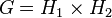# Congruence condition fails for number of normal subgroups of given prime power order

## Statement

It is possible to have a finite group$G$, and a prime power$p^r$ dividing the order of$G$, such that the number of normal subgroups of$G$ of order$p^r$ is a nonzero number that is not congruent to 1 mod$p$.

Note that any example must have$G$ as not being a finite$p$-group itself, because of the congruence condition on number of subgroups of given prime power order and the equivalence of definitions of universal congruence condition.

## Proof

Further information: direct product of A4 and Z4, direct product of A4 and V4

### Example of direct product of A4 and Z4

Let$G$ be the group direct product of A4 and Z4. This is a group of order 48 (GAP ID: (48,31)) obtained as the external direct product of alternating group:A4 (order 12) and cyclic group:Z4 (order 4). Suppose we denote:$G = H_1 \times H_2$

where$H_1$ is alternating group:A4 and$H_2$ is cyclic group:Z4.

Note that the order is of the form:$\! 48 = 2^4 \cdot 3$

The group has exactly two normal subgroups of order 4:

• The subgroup$K \times \{ e \}$ where$K$ is the subgroup in$H_1$ corresponding to V4 in A4, i.e., the subgroup$\{ (), (1,2)(3,4), (1,3)(2,4), (1,4)(2,3) \}$. This subgroup is isomorphic to a Klein four-group.
• The subgroup$\{ e \} \times H_2$. This subgroup is isomorphic to cyclic group:Z4.

Thus, the number of subgroups of order$2^2$ is 2, which is a nonzero number that is not congruent to 1 mod 2.

### Example of direct product of A4 and V4

Let$G$ be the group direct product of A4 and Z4. This is a group of order 48 (GAP ID: (48,31)) obtained as the external direct product of alternating group:A4 (order 12) and Klein four-group (order 4). Suppose we denote:$G = H_1 \times H_2$

where$H_1$ is alternating group:A4 and$H_2$ is Klein four-group.

Note that the order is of the form:$\! 48 = 2^4 \cdot 3$

The group has exactly two normal subgroups of order 4:

• The subgroup$K \times \{ e \}$ where$K$ is the subgroup in$H_1$ corresponding to V4 in A4, i.e., the subgroup$\{ (), (1,2)(3,4), (1,3)(2,4), (1,4)(2,3) \}$. This subgroup is isomorphic to a Klein four-group.
• The subgroup$\{ e \} \times H_2$. This subgroup is isomorphic to Klein four-group.

Thus, the number of subgroups of order$2^2$ is 2, which is a nonzero number that is not congruent to 1 mod 2.